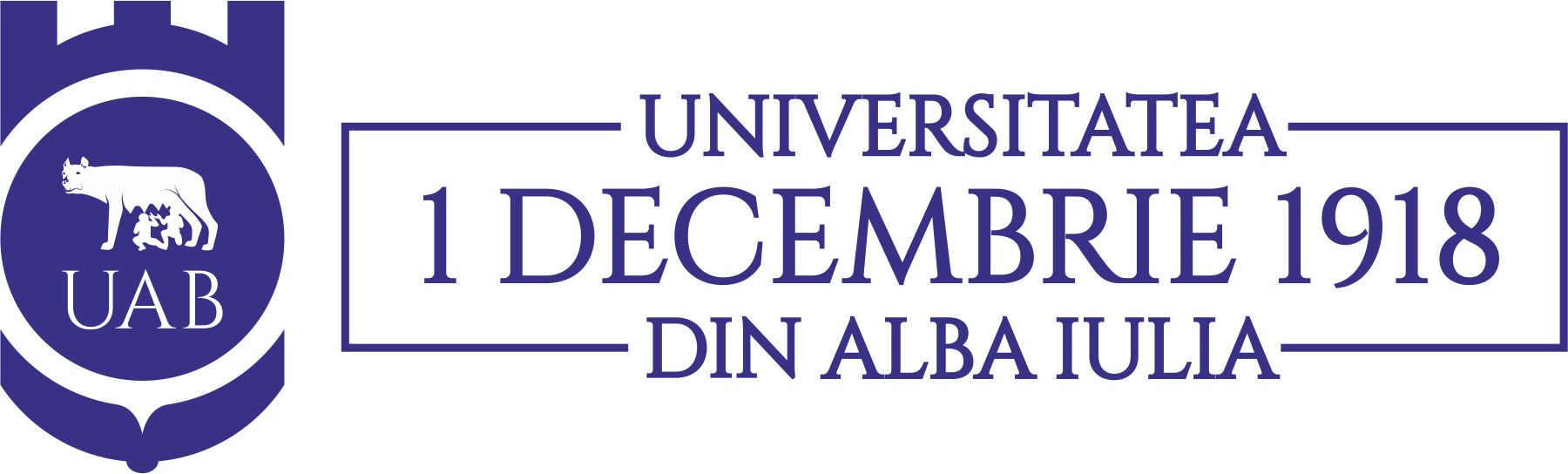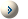Acta Universitatis Apulensis

Universitatea „1 Decembrie 1918” din Alba Iulia

Year 2014 / Acta / 40

SUMMARYA. Gilani - Generalized common fixed point for compatible mappings of type gamma  in complete fuzzy metric spacesH. Homei, B.N. Saray,  M. Lakestani - Numerical solution of nonlinear ordinary differential equations using Alpert multiwaveletsE. Abedi, R. Bahrami Ziabari - Slant submanifolds of a conformal Sasakian manifoldR. Atanasov, M. Budden, J. Lambert, K. Murphy, A. Penland - On Certain Induced Subgraphs of Paley GraphsM. Houas,  Z. Dahmani  - A Coupled System of Integro-Differential Equations Involving Riemann-Liouville Integral and Caputo DerivativeE. Deniz, Y. Ozkan  -- Subclasses of analytic functions defined by a new differential operatorG. Ozturk, B.K. Bayram, K. Arslan - Weak Biharmonic and Harmonic 1-Type Curves in Semi-Euclidean spaceH. Bulut, S. Tuluce Demiray, M. Kayhan - The approximate solutions of time-fractional diffusion equation by using Crank Nicholson methodT. Hamaizia, A. Aliouche - Fixed point theorems on two complete fuzzy metric spacesO. Oukacha, M. Aidene - Direct Method of solving optimal control problemsM. Alaeiyan, L. Pourmokhtar --   A classification of  cubic edge-transitive graphs of order $46p^{2}$M. El-Gamel, M.S. El-Azab, M. Fathy  --   The numerical solution of sixth order boundary value problems by the Legendre-Galerkin methodM.K. Aouf, A.O. Mostafa, A.Y. Lashin, B.M. Munassar - A study on general class of meromorphically univalent functionsM. Masal, N. Kuruoglu  -- On Spacelike Parallel p-Equidistant Ruled Surfaces in the Minkowski 3-SpaceN. Rajesh, P. Sudha --   A new type of covering dimensionP. Sharma, Om P. Ahuja, V.K. Gupta  --   Univalent Harmonic Functions with Domains Convex in Horizontal (Vertical) DirectionsK.I. Noor, Q.Z. Ahmad --  Subclasses of meromorphic univalent functionGeetha Balachandar(R. Geetha)  --  Fixed coefficients for a new subclass of uniformly spirallike functionsR.M. El-Ashwah,  D. Breaz  --  Special subclasses of harmonic functionsR.M. El-Ashwah  - Application of generalized   Hadamard product on special classes of analytic p-valent functionsR.M. El-Ashwah, M.K. Aouf, S.M. El-Deeb --  Some properties of certain subclasses of meromorphic multivalent functions of complex order defined by certain linear operatorM.K. Aouf, T.M. Seoudy, G.M. El-Hawsh - Some properties of generalized Hadamard  products for analytic functions with negative coefficientsU.C. De, Srimayee Samui - Quasi-conformal curvature tensor on generalized ($\kappa$, $\mu$)-contact metric manifoldsWei-ping Kuang  --  Lipschitz Estimate for Vector-valued Multilinear Commutator of Fractional Area Integral OperatorZ. Yucedag --  Infinitely many nontrivial solutions for nonlinear problems involving the $(p_{1}(x),p_{2}(x))$-Laplace operatorB. Laha, A. Bhattacharyya  --  Deformation of indefinite trans-Sasakian and  LSP Sasakian manifoldsS. Altinkaya, S. Yalcin --  Coefficient estimates for a certain subclass of analytic and bi-univalent functionsForeword by Michael H. Freedman Authors: Sotirios E. Louridas and Michael Th. Rassias - Book Review: Problem-Solving and Selected Topics in Euclidean Geometry: In the Spirit of the Mathematical OlympiadsA. Ebadian, R. Aghalary, M. A. Abolfathi  -- A fixed point approach to almost ternary homomorphisms and ternary derivations associated with the additive functional equation of n-Apollonius type in fuzzy ternary Banach algebrasA.O. Ogrenmis, M.Y. Yilmaz, M. Kulahci - Some Characterizations for Curves by the Help of Spherical Representations in the Galilean and Pseudo-Galilean Space